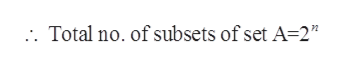How many subsets are there of {1, 2, 3, 4, 5, 6, 7, 8, 9, 10}? How many of these subsets are proper?

Question

How many subsets are there of {1, 2, 3, 4, 5, 6, 7, 8, 9, 10}? How many of these subsets are proper?

Step 1

Let the given set is A, having elements as

Step 2

Since the assumed set A has 10 elements namely {1,2,3,4,5,6,7,8,9,10}.

Therefore, the total number of subsets of set A=2n

Where, n=Total number of elements of set Ahelp_outlineImage Transcriptionclose. Total no. of subsets of set A-2" fullscreen
Step 3

Since, for set A the total number of elements is equal to 10.

i.e. n=10

So, Total number of sub...

Want to see the full answer?

See Solution

Want to see this answer and more?

Our solutions are written by experts, many with advanced degrees, and available 24/7

See Solution
Tagged in

Algebra